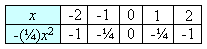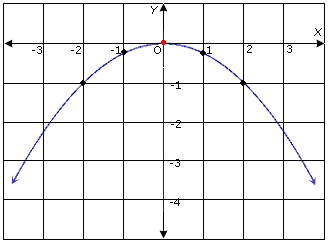Quadratic function is a function that can be described by an equation of the form f(x) = ax2 + bx + c, where a ≠ 0.

In a quadratic function, the greatest power of the variable is 2. The graph of a quadratic function is a parabola.

Quadratic equation: An equation in the standard form ax2 + bx + c = 0, where a ≠ 0 is called a quadratic equation.
Quadratic formula: A quadratic formula is the solution of a quadratic equation ax2 + bx + c = 0, where a ≠ 0, given by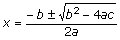Quadratic inequality: An inequality written in one of the forms y <ax2 + bx + c,
y ≥ ax2 + bx + cy ≤ ax2 + bx + c, or y > ax2 + bx + c is called a quadratic inequality.
Quadratic term: A term ax2 is the quadratic term in the equation f(x) = ax2 + bx + c

The following are few examples of quadratic functions.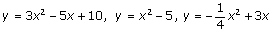Ques: Graph the quadratic function y = - (1/4)x2.Indicate whether the parabola opens up or down.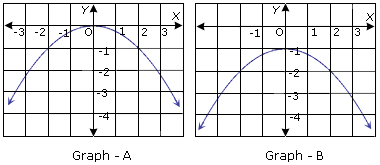Choices:

A. Graph-A; opens down
B. Graph-B; opens down